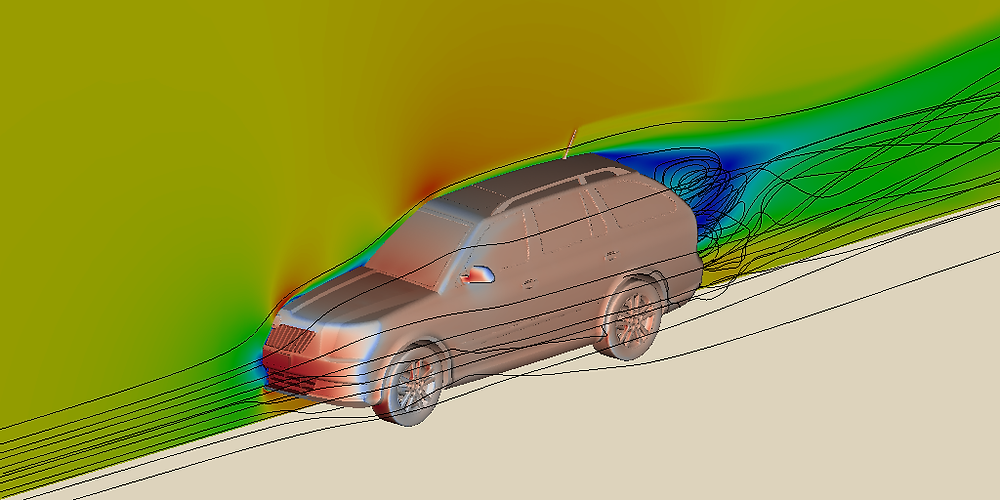Search
• Azuko

# Why is It Important to Use CFD Analysis?### What is CFD?

Gas and liquid flow behavior is quantified by partial differential equations representing conservation laws for mass, momentum, and energy. Computational fluid dynamics is a branch of fluid mechanics that uses numerical analysis and algorithms to solve fluid flows situations. High-performing computers are used to conduct the calculations required to simulate the interaction of liquids and gases with surfaces defined by boundary conditions.

CFD is based on the Navier-Stokes equations. Arising from applying Newton’s second law to fluid motion, together with the assumption that the stress in the fluid is the sum of a diffusing viscous term and a pressure term, these equations describe how the velocity, pressure, temperature, and density of a moving fluid are correlated.

The development of CFD has been closely associated with the evolution of high-speed computers.

### Brief History of CFD1922 – Basis of modern CFD and numerical meteorology made by Lewis Fry Richardson in a weather forecasting scheme using differential equations and finite differences.

1933 – Earliest numerical solution for flow past a cylinder developed by A. Thom.

1950 – First 24-hour weather forecast performed by the ENIAC modern computer.

1955 – Particle-in-cell simulation method for transient 2D fluid flow developed by Los Alamos National Lab.

1963 – Vorticity-stream-function method for 2D, transient, in compressible flow.

1965 – Marker-and-cell method for time-dependent viscous flow developed by Los Alamos National Lab.

1966 – Fluid-in-cell method developed for unsteady compressible flow problems.

1967 – First 3D model based on panels discretization published by Douglas Aircraft.

1968 – First lifting Panel Code (A230) described by Boeing Aircraft

1981 – 3D FLO57 code based on Euler equations for transonic flows

After 1981 – Many of the fundamental pieces of research that contributed to CFD 2D and 3D methods focused on air foil design and analysis. NASA research dedicated to the Navier–Stokes equations developed 2D codes ARC2D and 3D codes. These included codes like ARC3D, OVERFLOW, and CFL3D, which were the main sources for modern commercial CFD packages.

### Why is It Important to Use CFD Analysis?With a CFD analysis, we can understand the flow and heat transfer throughout a design process. The basic methodology for any engineering CFD analysis is based on a few procedures:

• Understanding flow model — Flow separations, transient effect, physical interactions;

• Proving assumed model — Experimental results validation, parametric studies, structural simulations;

• Model optimizing — Reducing pressure drops, flow homogenization, improving laminar and turbulent mixing.

Without numerical simulations of fluid flow, it is very difficult to imagine how: • Meteorologists can forecast the weather and warn of natural disasters;

• Vehicle designers can improve aerodynamic characteristics;

• Architects can design energy-saving and safe-living environments;

• Oil and gas engineers can design and maintain optimal pipes networks;

• Doctors can prevent and cure arterial diseases by computational hemodynamic.